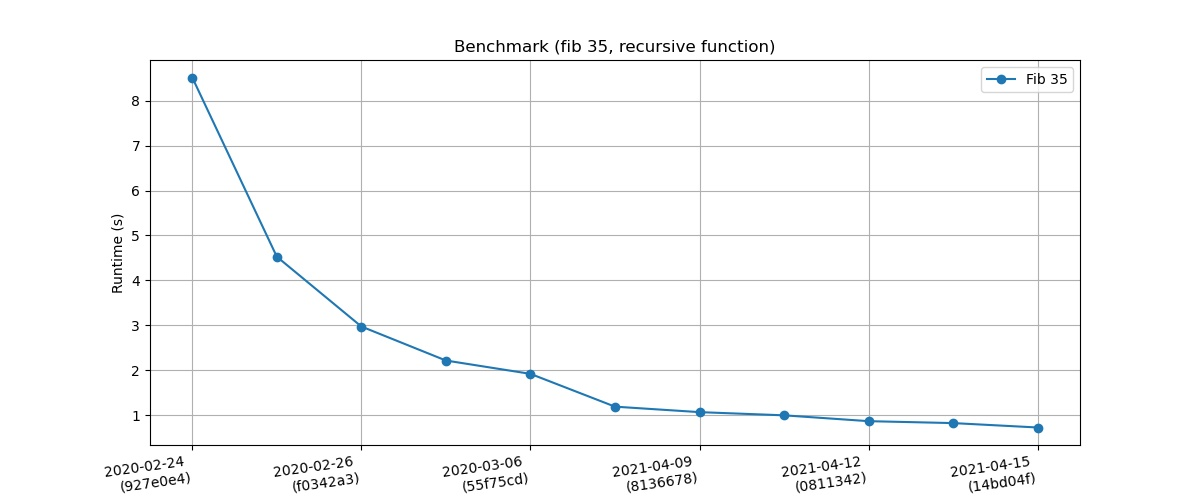{{ message }}

## dannyvankooten / monkey-c-monkey-do Public

C implementation of the Monkey programming language.

Switch branches/tags
Nothing to show

## Files

Failed to load latest commit information.
Type
Name
Commit time

Development of this interpreter continues here: dannyvankooten/pepper-lang

## C implementation of the Monkey programming language.Bytecode compiler and virtual machine for the Monkey programming language, written in C.

This is the result of going through the wonderful books Writing An Interpreter In Go and Writing A Compiler In Go, but using a different language instead to squeeze more learning opportunities out of it and force myself to really understand what's going on.

Since I like uselessly optimizing for performance, it runs quite fast for something that doesn't do JIT compilation.

### Syntax example

```// Variable declarations
let a = 5;
let b = 25;

// Reassign (previously declared) variables
a = a * 5;

// For loops
for (let i = 0; i < 10; i = i + 1) {
// i = 0 .. 9
}

// While loops
while (b > a) {
b = b - 5;
break;
}

// If statements
if (b == a || a == b) {
puts("b equals a!");
}

// Strings
let c = "Hello world";
c = str_split(c, " ");
type(c); // "ARRAY"
len(c); // 2

// Arrays
let d = [5, true, "Monkey"];
d; // 5
array_push(d, 10);
array_pop(d); // 10

// Functions
let fibonacci = fn(x) {
if (x < 2) {
return x;
}

return fibonacci(x - 1) + fibonacci(x - 2);
}

// Built-in functions
puts("35th fibonacci number is: ", fibonacci(35));
puts("Type of c = ", type(c));
puts("Length of c = ", len(c));
puts("Integer value of true = ", int(true));```

More examples can be found in the examples directory.

### Usage

Build Monkey interpreter (and REPL)

``````make
``````

Launch the REPL

``````./bin/monkey
``````

Interpret a Monkey script:

``````./bin/monkey examples/fib35.monkey
``````

Build & run tests

``````make check
``````

### Benchmarks

A benchmark to calculate the 35th fibonacci number using a recursive function is run on every commit through this Github action workflow.For fun, I ran the same algorithm expressed in some other interpreted languages on the same hardware (my laptop). This is how Monkey-C compares:

Language Time (s)
Node 15 0.21
Pypy 7.3 0.24
PHP 8.0 0.48
Monkey-C-Monkey-Do 0.72
Lua 5.4 0.72
Ruby 2.7 0.80
Python 3.9 1.91
PHP 5.6 2.72

C implementation of the Monkey programming language.

## Releases

No releases published

## Packages 0

No packages published